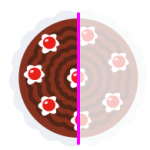Smartick is a fun way to learn math!# I want to learn about: Operations & Algebraic Thinking

Learn operations and algebraic thinking in elementary with Smartick. Exercises, theory, tutorials, and teaching resources about operations and algebraic thinking in elementary aged children. Here you will find posts about operations and algebraic thinking to make learning mathematics easier and more fun.

Oct23

## Learn and Practice the 11 and 12 Times Tables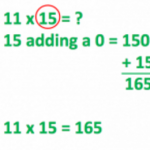In today’s post, we’re going to talk about 11 and 12 times tables. Schools generally teach the times tables from 1 to 10, and 11 and 12 times tables are something of an afterthought. However, the 11 and 12 times tables are gaining an increasing importance, and we’ll look at some strategies to help learn […]

Oct09

## Strategies for Solving Incomplete Horizontal Additions and SubtractionsIn today’s post, we’re going to take a look at some strategies for solving problems that involve incomplete horizontal additions and subtractions, using examples to help us. Acquiring mental calculation strategies is not always easy, since there’s no defined “best method” for everyone, and it can be frustrating if you don’t pick it up quickly. […]

Sep29

## Follow 8 Easy Steps to Factor a Number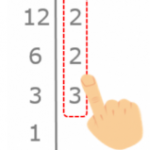In this entry, we’re going to explain how to break down or factor a number to its prime numbers step by step. Before we start anything, feel free to look at these entries where we explain what “factoring” means and what a prime number is so you can refresh your memory. Factorization How to factor […]

Sep27

## Learn about the Distributive Property of Multiplication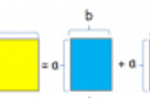In today’s entry, we are going to look at the distributive property of multiplication with respect to addition and subtraction. The distributive property is one of the properties of multiplication that is applied with respect to addition or subtraction. This property tells us that if we add or subtract several numbers and we multiply the […]

Sep25

## The Associative Property in Addition and Multiplication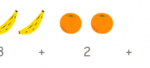Addition and multiplication both use the associative property, while subtraction and division do not. We’re going to to get up close with each situation to get a better idea. The associative property in Addition ♥ Addition indeed has the associative property. Whatever numbers a, b, and c may be, they always end up the same: […]

Sep20

## Introduction to Equations with an Example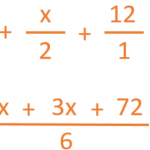In today’s post we’re going to look at the concept of equations. To start, we’re going to take a look at this situation: “One third of Cecilia’s flowerpots contain daisies, half of them are full of pansies, and the other twelve contain poppies. How many flowerpots does Cecilia have?” Think about it, and see if […]

Sep13

## Understanding Multiplication Problems with Examples

What needs to be done in order to solve multiplication problems? Isn’t it enough to just multiply the numbers that we’re given? No! Because… What’s more important is UNDERSTANDING the multiplication problems. This is why we’re bringing you some multiplication problems today where YOU WON’T NEED TO USE MULTIPLICATION, but rather, TO THINK CRITICALLY. Ready? […]

Sep08

## Review the Different Properties of MultiplicationSometimes multiplying can be complicated but knowing the tables and properties of multiplication, we can make this task much easier… let’s get to it! There are three properties of multiplication: commutative, associative, and distributive. Commutative Property It states that the order of the factors does not change the product, that is, it does not matter if […]

Sep01

## How to Solve a Subtraction Problem with Regrouping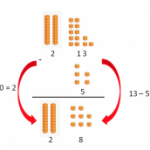Do you know how to solve a subtraction problem with regrouping? Do you know how regrouping is done and why? Today we are going to review how to subtract by regrouping and understand why it is done this way. So that we can picture it better, we are going to do a subtraction with regrouping […]

Aug28

## Multiplying Numbers Followed by Zeros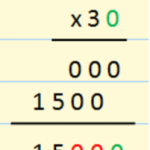In today’s post, we’re going to learn how to multiply numbers that are followed by zeros. You could go about multiplying them like any other number, but we have a trick to teach you, and it’s easy to learn! The most important thing when you learn a trick is to remember why is works the […]

Aug16

## Review Factoring with Examples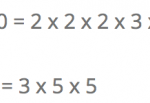Hello again! Do you know what factoring is? Do you know what it is used for? In this post we will answer these questions. How to Factor The factorization of a number is performed by writing the number as the multiplication of all its prime factors. Example: 12 = 2 x 2 x 3 You can […]